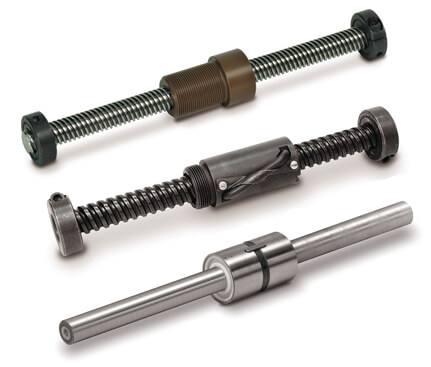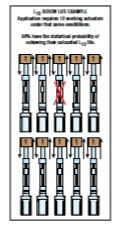# How to calculate electric linear actuator service life

By Ryan Klemetson on April 21, 2020“How long will it last?” Every machine design engineer gets asked this question and has to calculate the anticipated life of their machine—life that’s based on machine components including electric linear actuators. Also, expected service life is a factor engineers consider when they’re evaluating competing components.

Calculating electric linear actuator life can be straight-forward for ball screw and roller screw actuators. You use the L10 life formula for ball bearings. The life calculation for electric linear actuators focuses on these rolling elements because the screw/nut combination is a screw-driven electric linear actuator’s critical moving component.

Here’s a summary of the method. For a thorough explanation, download our Actuator Life Guide.

## Definitions

Here are definitions of critical terms you’ll need to know.

Dynamic Load Rating (DLR): This number is usually provided by the manufacturer and is represented by the letter C. It represents a constantload under which a ball bearing device will achieve 1,000,000 revolutions (rotations) of rated life.

Constant load: This is a load that remains unchanged along the full working cycle.

Equivalent Dynamic Load: Equivalent dynamic load is a dynamic load acting on the screw which, if applied constantly, would have the same effect on screw life as the combined actual loads. When an application has a varying load, you have to calculate the equivalent dynamic load or Pe. In the case of constant load, equivalent load = actual load.

L10 (or B10) Life: L10 is a calculation of life at which 10% of bearings in the same application can be expected to fail due to classic fatigue failure. L10 life for a group of identical screw actuators operating under the same conditions is thenumber of revolutions (or distance of travel) which 90% of these actuators have the statistical probability to achieve.

This calculation provides a theoretical life estimate based on a collection of statistics. It’s not a guarantee of performance but a guide for expected life.

## How to calculate L10 life with a constant load

To estimate ball screw or roller screw actuator life with a constant load, the underlying formula is:

## L10 = (C/Pe)3 x l

C = Dynamic load rating (lbf) or (N)

Pe = Equivalent load (lbf) or (N)

l = Screw lead (in/rev) or (mm/rev)

For example, in a case where:

C = 10,000 lbf

Pe = 5,000 lbf

l = 5 mm

## How to calculate L10 life with a varying load

When a load varies during the working cycle you first have to calculate the equivalent dynamic load (Pe) using this formula:

## Pe = 3 √{ [L1(P1)3+L2(P2 )3+L3(P3 )3+Ln(Pn )3]/L}

Pe = Equivalent load (lbs) or (N)

Pn = Each increment at different load (lbs) or (N)

L = Total distance traveled per cycle (extend + retract stroke) (L = L1 + L2 + L3 + Ln)

Ln = Each increment of stroke (in) or (mm) at different load

Then you can use the calculated Pe in the life calculation formula:

## How to calculate estimated life in timeThe above calculations are helpful; however, machine buyers will probably want to know “how long it will last” in terms of minutes, hours, days, or years. For electric linear actuators, here’s what you need to know for this calculation:

L10        life calculation in distance

L            total distance traveled per cycle (extend + retract stroke)

CpM      Number of cycles per minute

HpD      Number of hours operated per day

DpY       Number of days of operation per year

Put that information into one of these formulas:

## (L10 / L) / [(CpM) X 60 min/hr X (HpD)]

This gives you the estimated life of the ball screw or roller screw actuator in units of time.